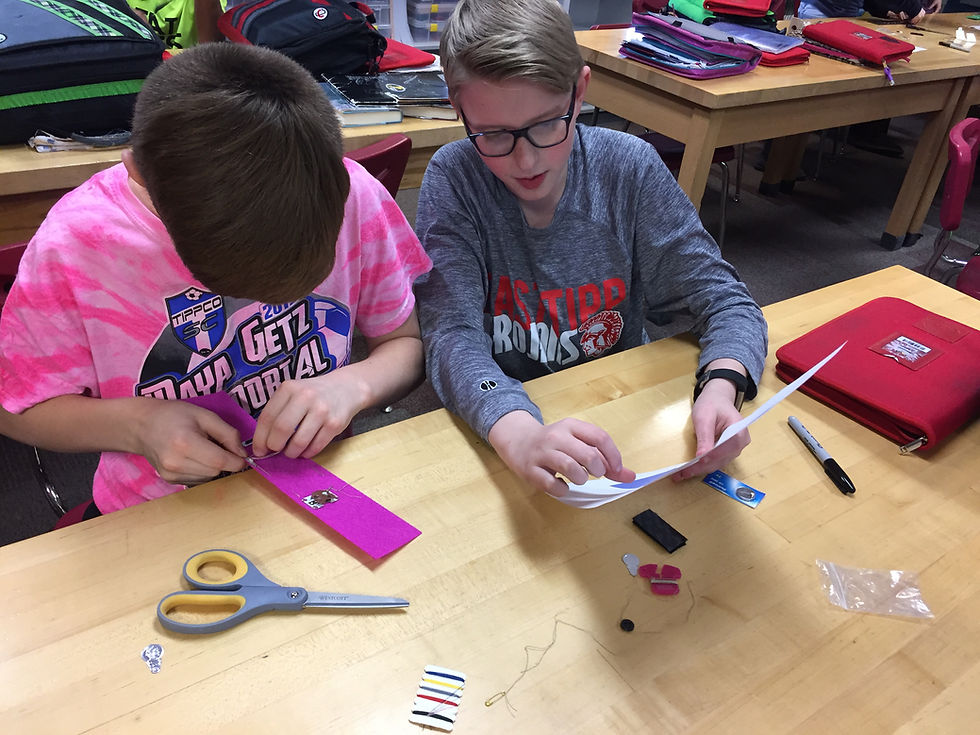top of pageEngineering Knowledge:
Engineering Sciences## Engineering Performance Matrix

Mechanics of Materials concerns the mechanical behavior of deformable bodies when they are subjected to stresses, loads, and other external forces. This concept is important to Engineering Literacy, as it is the basis on which engineers select materials and modify their forms to create mechanical devices and systems. For example, the application of this knowledge enables professionals to predict structural failure by using Stress-Strain analyses and Young’s modulus to evaluate an object's deformation resulting from applied loads.

## Performance Goal for High School Learners

I, when appropriate, draw upon the knowledge of the Mechanics of Materials, such as (a) stress types and transformations, (b) material characteristics, (c) stress-strain analysis, (d) material deformations, (e) material equations, (f)phase diagram, (g)Mohr’s circle, and (h)Young’s modulus, to analyze the properties, compositions, and behaviors of available, or needed, materials to solve problems in a manner that is analytical, predictive, repeatable, and practical.

## STRESS TYPES & TRANSFORMATIONS

I can distinguish between normal and sheer stress and their effects on materials.

## MATERIAL CHARACTERISTICS,PROPERTIES, & COMPOSITION

I can list various materials that are commonly used in mechanical systems and describe how materials are characterized and categorized.

I can explain the objectives and basic methods of stress transformations.

I can explain the basic characteristics, properties, and composition of given materials.

I can analyze the stress state of a material through calculating the magnitudes of normal stress and shear stress components.

I determine and justify which materials are most appropriate for my design.

## STRESS-STRAIN ANALYSIS

I can describe the relationship between stress and strain in materials.

I can interpret a given stress-strain curve.

I can analyze stress-strain behavior of a certain material with a given stress-strain curve.

## MATERIAL DEFORMATIONS

I can describe different types of material deformations and their effects on mechanical systems.

I can explain different types of material deformation in terms of stress and strain.

I can analyze the deformation of a certain material with its stress-strain curve.

## MATERIAL EQUATIONS

I can describe the applications of the equations in designing mechanical systems.

I can search for the equations and explain each variable of the equations.

I can apply appropriate equations to analyze or model the behavior of the materials to be used for my design.

## PHASE DIAGRAM

I can describe the basic components and applications of phase diagrams.

I can explain the thermodynamically distinct phases of a certain material with its phase diagram.

I can apply phase diagrams to analyze and model the thermodynamically distinct phases of the materials to be used for my design.

## MOHR’S CIRCLE

I can describe the function and basic elements of Mohr’s circle in a stress analysis.

I can draw Mohr’s circle for the stress analysis on a material.

I can analyze the stress state of a material through using equations of Mohr’s circle.

## YOUNG’S MODULUS

I can describe Young’s modulus in terms of stiffness and elasticity of solid materials.

I can explain Young’s modulus in terms of its variables (force, cross-sectional area, and length of the object).

I can calculate Young’s modulus to analyze the stiffness of a solid material and elastic deformation.

bottom of page